# Tickets

On Monday, 33 tickets were sold. Every next day, twice as many as the previous day. How many tickets were sold on Friday and how many totally from Monday to Friday?

Result

l5 =  528
s =  1023

#### Solution:Leave us a comment of example and its solution (i.e. if it is still somewhat unclear...):

Showing 0 comments:Be the first to comment!## Next similar examples:

1. Word problem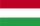395 students were on holiday in Hungary. 110 bought pizza, chips 21 pupils, 26 pupils bought soda, butter 18 pupils, 12 pupils fruits, vegetables 29 students. How much pay for all the food, if every meal cost 5.5 euros?
2. The percentages in practiceIf every tenth apple on the tree is rotten it can be expressed by percentages: 10% of the apples on the tree is rotten. Tell percent using the following information: a. in June rained 6 days b, increase worker pay 500 euros to 50 euros c, grabbed 21 fro
3. Down syndromeDown syndrome is one of the serious diseases caused by a gene mutation. Down syndrome occurs in approximately every 550-born child. Express the incidence of Down's syndrome in newborns at per mille.
4. Apples 2James has 13 apples. He has 30 percent more apples than Sam. How many apples has Sam?
5. Percents - easyHow many percent is 432 out of 434?
6. Combine / add termCombine like terms 4c+c-7c
7. Percentages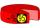Expressed as a percentage:
8. The squareThe square root of 25 times the square root of 81 is what number?
9. Double percent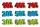What is 80% of 60% of 2800?
10. Decimal expansion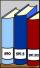Calculate: 2 . 1 + 0 . 10 + 7 . 10000 + 4 . 1000 + 6 . 100 + 0 . 100000 =
11. ClassIn a class are 32 pupils. Of these are 8 boys. What percentage of girls are in the class?
12. Average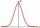Average of 7 numbers is 65. What is its sum?
13. Conference148 is the total number of employees. The conference was attended by 22 employees. How much is it in percent?
14. Watching TVOne evening 2/3 students watch TV. Of those students, 3/8 watched a reality show. Of the students that watched the show, 1/4 of them recorded it. What fraction of the students watched and recorded reality tv.
15. Unknown numberIdentify unknown number which 1/5 is 40 greater than one tenth of that number.
16. Hexadecimal number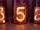What will be hexadecimal number 303 as decimal number?
17. EquationSolve the equation: 1/2-2/8 = 1/10; Write the result as a decimal number.